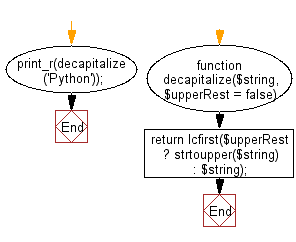﻿ PHP Exercise: Decapitalize the first letter of the string and then adds it with rest of the string - w3resource

# PHP Exercises: Decapitalize the first letter of the string and then adds it with rest of the string

## PHP: Exercise-97 with Solution

Write a PHP program to decapitalize the first letter of the string and then adds it with rest of the string.

Sample Solution: -

PHP Code:

``````<?php
//Licence: https://bit.ly/2CFA5XY

function decapitalize(\$string, \$upperRest = false)
{
return lcfirst(\$upperRest ? strtoupper(\$string) : \$string);
}
print_r(decapitalize('Python'));

?>
```
```

Sample Output:

```python
```

Flowchart:PHP Code Editor:

Have another way to solve this solution? Contribute your code (and comments) through Disqus.

What is the difficulty level of this exercise?

Test your Programming skills with w3resource's quiz.

﻿

## PHP: Tips of the Day

Mutates the original array to filter out the values specified

Example:

```<?php
function tips_pull(&\$items, ...\$params)
{
\$items = array_values(array_diff(\$items, \$params));
return \$items;
}

\$items = ['x', 'y', 'z', 'x', 'y', 'z'];
print_r(tips_pull(\$items, 'y', 'z'));
?>
```

Output:

```Array
(
 => x
 => x
)
```